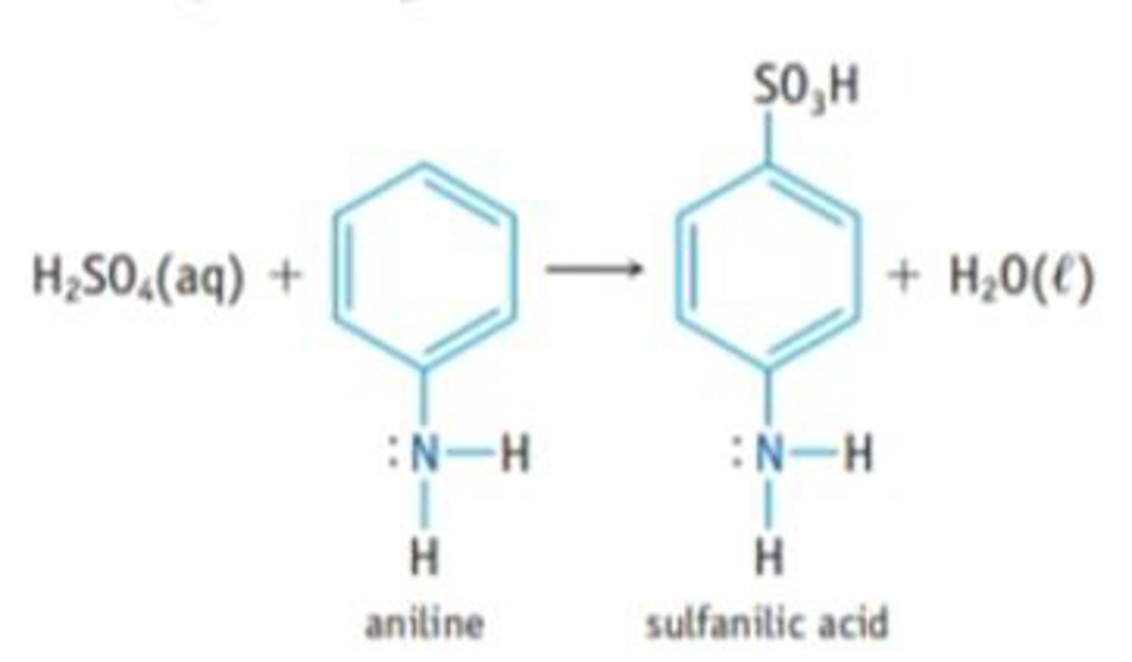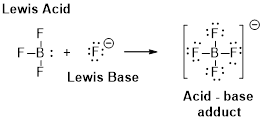Chapter 16, Problem 117IL

Chapter
Section
Textbook Problem

Sulfanilic acid, which is used in making dyes, is made by reacting aniline with sulfuric acid.(a) Is aniline a Brønsted base, a Lewis base, or both? Explain, using its possible reactions with HCl, BF3, or other acid. (b) Sulfanilic acid has a pKa value of 3.23. The sodium salt of the acid, Na(H2NC6H4SO3), is quite soluble in water. If you dissolve 1.25 g of the salt in water to give 125 ml, of solution, what is the pH of the solution?

(a)

Interpretation Introduction

Interpretation:

Aniline has to be idetentified whether a bronsted base or a Lewis base or both.

Concept Introduction:

Bronsted –Lowry definitions:

A Bronsted –Lowry acid is a proton donor, it donates a hydrogen ion, H+

A Bronsted-Lowry base is a proton acceptor, it accepts a hydrogen ion H+.Lewis definition:

A Lewis acid is a substance that can accept and share an electron pair.

A Lewis base is a substance that can donate and share an electron pair.Explanation

Aniline is a bronsted base because the lone pair of electrons on the nitrogen atom can accept a hydrogen ion, it reacts with a strong acid like HCl, the reaction is completely straight forward, a solution of salt is formed-phenylammonium chloride.

C6H5NH2(aq)+HCl(aq)C6H5NH3+Cl(aq)

Aniline is also acted as a Lewis base because the Nitrogen atom has a lone pair of the electron that can donate to an empty orbital of other species, BF3 contains an empty orbital can accept a lone pair of an electron from Nitrogen atom of Aniline,

C6H5NH2(aq)+BF3

(b)

Interpretation Introduction

Interpretation:

The pH of the solution has to be calculated when solution containing Sodium salt of a Sulfanilic acid.

Concept Introduction:

The pOH is a measure of Hydroxide ion concentration and pH is a measure of Hydrogen ion concentration.

pOH=log[OH] (1)

The sum of pH and pOH is equal to 14 at 25°C.

pH+pOH=14 (2)

Still sussing out bartleby?

Check out a sample textbook solution.

See a sample solution

The Solution to Your Study Problems

Bartleby provides explanations to thousands of textbook problems written by our experts, many with advanced degrees!

Get Started